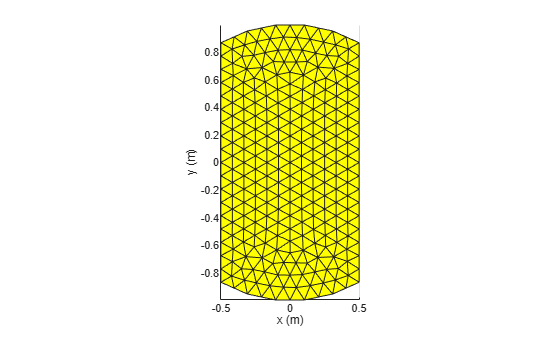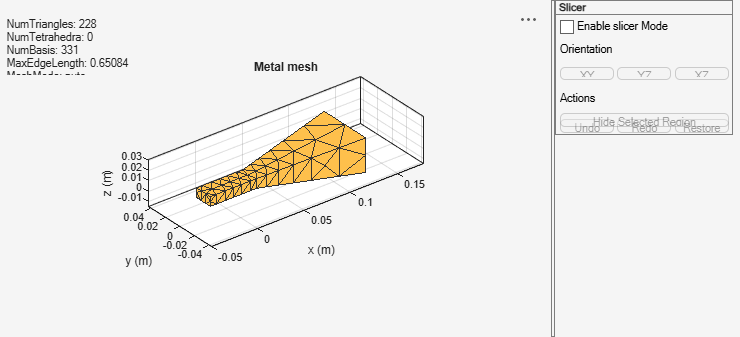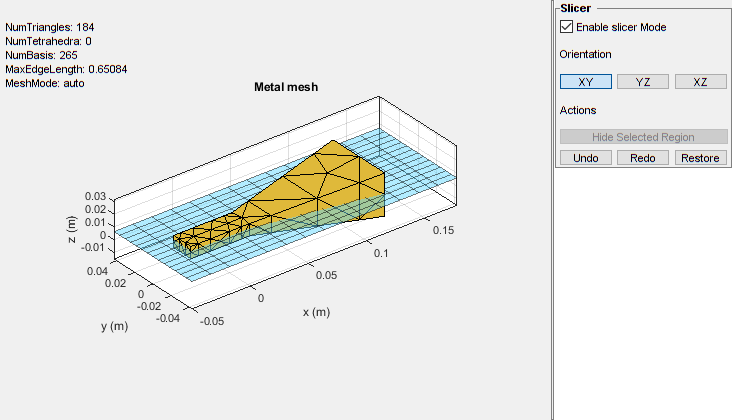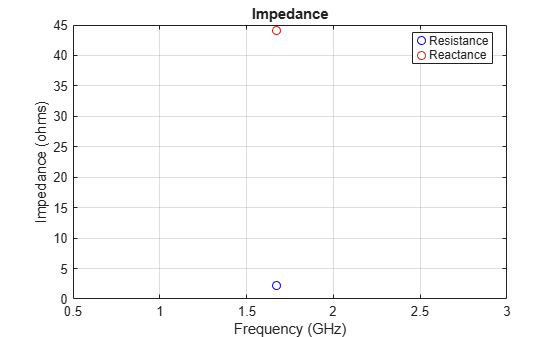# mesh

Mesh properties of metal, dielectric antenna, or array structure

## Syntax

``mesh(object)``
``mesh(object,MaxEdgeLength=Value)``
``mesh(___,Name=Value)``
``meshdata = mesh(___)``

## Description

example

````mesh(object)` generates a plot of meshed antenna, array, custom geometric shape, or a platform. You must run any analysis (impedance, current, charge, pattern etc.) on the antenna or array prior to generating the mesh plot. Here, the `MeshMode` is `"auto"`.```

example

````mesh(object,MaxEdgeLength=Value)` generates a plot of meshed antenna, array, custom geometric shape, or a platform. Here, the `MeshMode` is `"manual"` and you must specify the maximum edge length for the mesh to generate the plot. You do not need to run any analysis (impedance, current, charge, pattern etc.) on the antenna or array prior to generating the mesh plot.```

example

````mesh(___,Name=Value)` generates a plot of meshed antenna, array, custom geometric shape, or a platform with additional properties specified using one or more Name-Value Arguments.```

example

````meshdata = mesh(___)` returns a `MeshReader` object with read-only mesh properties. Use this syntax to see the details about the mesh parameters and quality.```

## Examples

collapse all

Create a top-hat monopole antenna and run impedance analysis on it.

```h = monopoleTopHat; i = impedance(h,75e6)```
```i = 2.4847e+02 + 5.9963e+02i ```

VIew the antenna mesh.

`mesh(h)`View the mesh properties.

`m = mesh(h)`
```m = MeshReader with properties: Points: [3x92 double] Triangles: [4x144 double] Tetrahedra: [] MaxEdgeLength: 0.4295 MinEdgeLength: 0.3221 GrowthRate: 0.9500 MinimumMeshQuality: 0.0660 MeshMode: 'auto' ```

Radiation Pattern of Microstrip Patch Antenna

Create a microstrip patch antenna using 'FR4' as the dielectric substrate.

```d = dielectric("FR4"); pm = patchMicrostrip(Length=75e-3,Width=37e-3,... GroundPlaneLength=120e-3,GroundPlaneWidth=120e-3,... Substrate=d); show(pm)```Plot the radiation pattern of the antenna at a frequency of 1.67 GHz.

```figure pattern(pm,1.67e9)```Mesh the whole antenna.

```figure mesh(pm)```Mesh only the dielectric surface of the antenna.

```figure mesh(pm,View="dielectric surface")```Create a rectangular and circular shape, intersect them and mesh at a wavelength of 2 m.

```r = antenna.Rectangle; c = antenna.Circle; p = r&c; mesh(p,2);```Create a default horn antenna. Mesh the structure with the `Slicer` argument set to "`on"`.

```ant = horn; z = impedance(ant,70e6); mesh(ant,Slicer="on")```Select Enable slicer Mode. Then select a plane for the slice under Orientation.Click on the plot and select a region to hide.Click Hide Selected Region to view the desired slice.This example shows how to read mesh parameters of a microstrip patch antenna and view the metal, dielectric, and complete mesh.

Create Microstrip Patch Antenna

Create a microstrip patch antenna with copper conductor and FR4 PCB substrate. Run impedance analysis on this antenna at 1.67 GHz to automatically generate the mesh.

```p = patchMicrostrip(Conductor=metal("Copper"),Substrate=dielectric("FR4")); figure impedance(p,1.67e9);```Read the mesh parameters such as number of points, triangles, and terahedra, maximum and minimum edge length, mesh growth rate, and quality using the `MeshReader` object.

`m = mesh(p)`
```m = MeshReader with properties: Points: [3x1644 double] Triangles: [4x2014 double] Tetrahedra: [4x4620 double] MaxEdgeLength: 0.0044 MinEdgeLength: 2.2012e-04 GrowthRate: 0.9500 MinimumMeshQuality: 0.0803 MeshMode: 'auto' ```

View Mesh of Antenna

View the metal mesh.

```figure showMetalMesh(m)```View the dielectric surface mesh.

```figure showDielectricSurfaceMesh(m)```View the dielectric volume mesh.

```figure showDielectricVolumeMesh(m)```View the overall mesh.

```figure showMeshAll(m)```## Input Arguments

collapse all

Antenna or array from the catalog, custom antenna or array created using 2-D and 3-D objects from Custom 2-D and 3-D Antenna catalog, `installedAntenna` or large `platform`, `pcbStack` antenna or array, specified as an object.

Example: `dipole`

Example: `conformalArray`

Example: `antenna.Rectangle`

Example: `shape.Sphere`

Example: `customAntenna`

Example: `installedAntenna`

Example: `pcbStack`

### Name-Value Arguments

Specify optional pairs of arguments as `Name1=Value1,...,NameN=ValueN`, where `Name` is the argument name and `Value` is the corresponding value. Name-value arguments must appear after other arguments, but the order of the pairs does not matter.

Example: `MaxEdgeLength=0.1`

Before R2021a, use commas to separate each name and value, and enclose `Name` in quotes.

Example: `'MaxEdgeLength',0.1`

Maximum edge length of triangles in the mesh, specified as a scalar in meters.

Example: `0.01`

Data Types: `double`

Smallest edge length of triangles in the mesh, specified as a positive scalar in meters.

Example: `2e-3`

Data Types: `double`

Gradation in the triangle sizes of the mesh, specified as a scalar in the range (0,1).

Example: `GrowthRate=0.7` states that the growth rate of the mesh is 70 percent.

Data Types: `double`

Choice of the antenna or array element layer to view the mesh plot, specified as a string. Choose:

• `"all"` to view the mesh of the whole antenna or array.

• `"metal"` to view the metal layer mesh of the antenna or array.

• `"dielectric surface"` to view the boundary triangle mesh of the dielectric.

• `"dielectric volume"` to view the tetrahedral volume mesh of the dielectric.

Example: `"metal"`

Data Types: `string`

Option to enable or disable plot interactivity, specified as `"on"` or `"off"`, or as numeric or logical `1(true)` or `0(false)`. Set this argument to `1` or `"on"` to open the plot with the slicer panel, and to slice and view the desired cross section of the plot along the xy-, yz-, and xz- planes. Set this argument to `0` or `"off"` to open the plot without the slicer panel.

Example: `"on"`

Data Types: `double` | `logical` | `string`

## Output Arguments

collapse all

The mesh properties such as number of triangles, tetrahedra, and basis functions in the mesh, maximum and minimum edge lengths, and growth rate stored in a `MeshReader` object.

## Version History

Introduced in R2015a